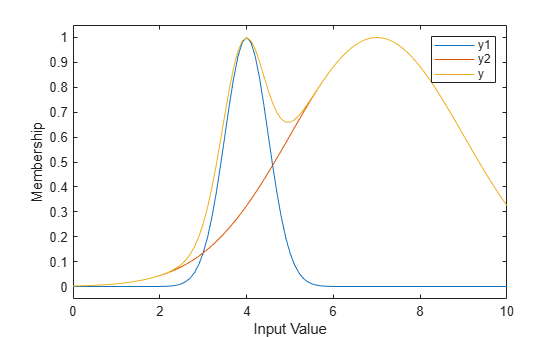Documentation

# probor

Probabilistic OR

## Syntax

```y = probor(x)
```

## Description

`y = probor(x)` returns the probabilistic OR (also known as the algebraic sum) of the columns of `x`. if x has two rows such that ```x = [a; b]```, then `y = a + b - ab`. If `x` has only one row, then `y = x`.

## Examples

collapse all

```x = 0:0.1:10; y1 = gaussmf(x,[0.5 4]); y2 = gaussmf(x,[2 7]); yy = probor([y1;y2]); plot(x,[y1;y2;yy]) legend('y1','y2','yy') ylim([-0.05 1.05])```